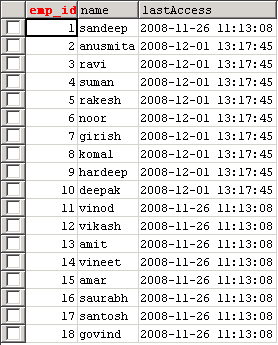# PHP SQL Left Join

This Example illustrates how to use left join clause in the sql query. The left join return all rows from the left table, even if there are no matches in the right table.

This Example illustrates how to use left join clause in the sql query. The left join return all rows from the left table, even if there are no matches in the right table.

# PHP SQL Left Join

This Example illustrates how to use left join clause in the sql query. The left join return all rows from the left table, even if there are no matches in the right table.

In this example left join is used to join the two table data according to the "emp" table id. The fetched data displays in the table format on the browser. We use "emp" table and "message" table and find the emp.name from emp table and find the message.messages from message table where emp.emp_id and message.emp_id both are equal. The fetched result display on the browser as below and the complete source code is available below for download or copy the code as per your need.

The "LEFT JOIN" keyword is used for join the table data.

Table: empTable: MessageSource Code of leftJoin.php

 ```
NameMessage
" . \$row['name'] . "" . \$row['messages'] . "
";     while (\$row = mysql_fetch_array(\$result)) {       echo "";       echo "";       echo "";       echo "";     }   echo "";      mysql_close(\$con); ?>```

Output: